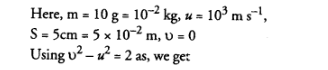# Using second law of motion, derive the relation`
Question:

Using second law of motion, derive the relation between force and acceleration. A bullet of $10 \mathrm{~g}$ strikes a sand-bag at a speed of $10^{3} \mathrm{~ms}^{-1}$ and gets embedded after travelling $5 \mathrm{~cm}$. Calculate

(i) the resistive force exerted by the sand on the bullet

(ii) the time taken by the bullet to come to rest.

Solution:

According to this law, the change in momentum of a body per unit time (i.e. rate of change of momentum)

is directly proportional to the unbalanced force acting on the body and the change in momentum takes

place in the direction of the unbalanced force on the body.

Consider a body of mass moving with initial velocity Let a force acts on the body for time so that the

velocity of the body after time is.

Initial momentum of the body, $p_{i}=m u$

Final momentum of the body, $p_{f}=m v$

Now, change in momentum of the body $=p_{f}-p_{i}=m v-m u=m(v-u)$

$\therefore \quad$ Rate of change of momentum $=\frac{\text { Change of momentum }}{\text { Time taken }}$

$=\frac{m(v-u)}{t}$

According to the definition of Newton's second law of motion Force applied $\propto$ rate of change of momentum

Or

$\mathrm{F} \propto \frac{m(v-u)}{t}$ $\ldots(i)$

Since

$v=u+a t \quad$ or $\quad\left(\frac{v-u}{t}\right)=a$

Therefore, eqn. $(i)$ can be written as

$\therefore \quad \quad \mathrm{F} \propto m a$

Or        $\quad F=k m a$$\ldots(i i)$

where $k$ is constant of proportionality

If

$F=1$ unit

$m=1$ unit

$a=1$ unit

and

Then from eqn. (ii),

$1=k \quad$ or $\quad k=1$

Put this value of $k=1$ in eqn. (2), we get

$\mathrm{F}=m a$.......(1)

Thus, force acting on the body is directly proportional to (i) its mass (m) and (ii) its acceleration (a).

Eqn. (1) gives the mathematical form of Newtons second law of motion. The force given by eqn (1) acts on

the body.

Newton's second law of motion in vector form

We know, force (F) and acceleration ( a) are vector quantities, whereas mass ( $\mathrm{m}$ ) is a scalar quantity.

Therefore, Newton's second law of motion can be written in vector form as

$\overrightarrow{\mathrm{F}}=m \vec{a}$

This relation shows that the direction of force applied on the body is same as that of the acceleration$a=\frac{v^{2}-u^{2}}{2 s}$

$=\frac{0-10^{6}}{2 \times 5 \times 10^{-2}}=-10^{7} \mathrm{~m} \mathrm{~s}^{-2}$

(i) Resistive force,

$\mathrm{F}=m a=-10^{-2} \times 10^{7}=-10^{5} \mathrm{~N}$

(ii) Using $\mathrm{v}=u+a t$, we get

$t=\frac{v-u}{a}=\frac{0-10^{3}}{-10^{7}}=10^{-4} \mathrm{~s}$

produced in the body.